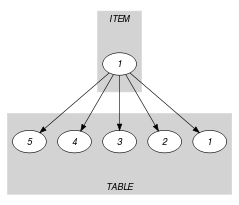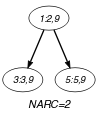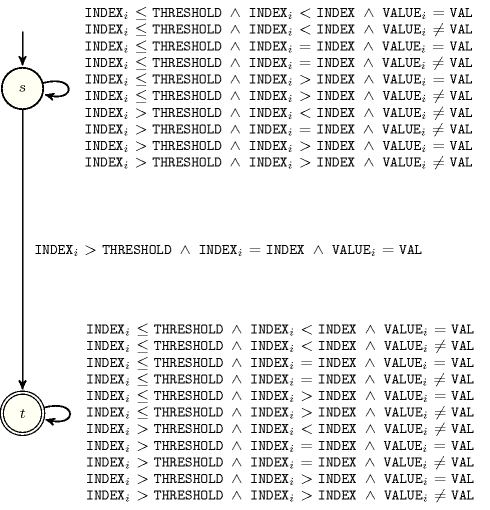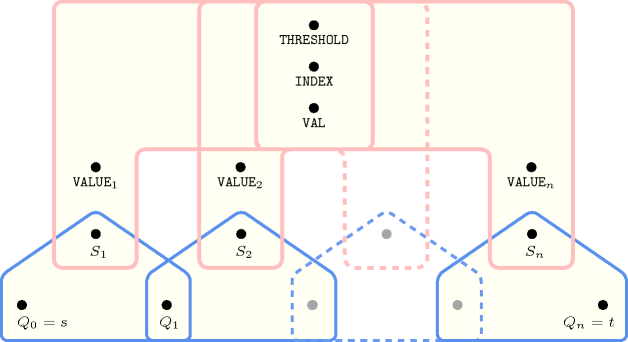## 5.275. next_element

Origin

N. Beldiceanu

Constraint

$\mathrm{𝚗𝚎𝚡𝚝}_\mathrm{𝚎𝚕𝚎𝚖𝚎𝚗𝚝}\left(\mathrm{𝚃𝙷𝚁𝙴𝚂𝙷𝙾𝙻𝙳},\mathrm{𝙸𝙽𝙳𝙴𝚇},\mathrm{𝚃𝙰𝙱𝙻𝙴},\mathrm{𝚅𝙰𝙻}\right)$

Arguments
 $\mathrm{𝚃𝙷𝚁𝙴𝚂𝙷𝙾𝙻𝙳}$ $\mathrm{𝚍𝚟𝚊𝚛}$ $\mathrm{𝙸𝙽𝙳𝙴𝚇}$ $\mathrm{𝚍𝚟𝚊𝚛}$ $\mathrm{𝚃𝙰𝙱𝙻𝙴}$ $\mathrm{𝚌𝚘𝚕𝚕𝚎𝚌𝚝𝚒𝚘𝚗}\left(\mathrm{𝚒𝚗𝚍𝚎𝚡}-\mathrm{𝚒𝚗𝚝},\mathrm{𝚟𝚊𝚕𝚞𝚎}-\mathrm{𝚍𝚟𝚊𝚛}\right)$ $\mathrm{𝚅𝙰𝙻}$ $\mathrm{𝚍𝚟𝚊𝚛}$
Restrictions
 $\mathrm{𝙸𝙽𝙳𝙴𝚇}\ge 1$ $\mathrm{𝙸𝙽𝙳𝙴𝚇}\le |\mathrm{𝚃𝙰𝙱𝙻𝙴}|$ $\mathrm{𝚃𝙷𝚁𝙴𝚂𝙷𝙾𝙻𝙳}<\mathrm{𝙸𝙽𝙳𝙴𝚇}$ $\mathrm{𝚛𝚎𝚚𝚞𝚒𝚛𝚎𝚍}$$\left(\mathrm{𝚃𝙰𝙱𝙻𝙴},\left[\mathrm{𝚒𝚗𝚍𝚎𝚡},\mathrm{𝚟𝚊𝚕𝚞𝚎}\right]\right)$ $|\mathrm{𝚃𝙰𝙱𝙻𝙴}|>0$ $\mathrm{𝚃𝙰𝙱𝙻𝙴}.\mathrm{𝚒𝚗𝚍𝚎𝚡}\ge 1$ $\mathrm{𝚃𝙰𝙱𝙻𝙴}.\mathrm{𝚒𝚗𝚍𝚎𝚡}\le |\mathrm{𝚃𝙰𝙱𝙻𝙴}|$ $\mathrm{𝚍𝚒𝚜𝚝𝚒𝚗𝚌𝚝}$$\left(\mathrm{𝚃𝙰𝙱𝙻𝙴},\mathrm{𝚒𝚗𝚍𝚎𝚡}\right)$
Purpose

$\mathrm{𝙸𝙽𝙳𝙴𝚇}$ is the smallest entry of $\mathrm{𝚃𝙰𝙱𝙻𝙴}$ strictly greater than $\mathrm{𝚃𝙷𝚁𝙴𝚂𝙷𝙾𝙻𝙳}$ containing value $\mathrm{𝚅𝙰𝙻}$.

Example
$\left(\begin{array}{c}2,3,〈\begin{array}{cc}\mathrm{𝚒𝚗𝚍𝚎𝚡}-1\hfill & \mathrm{𝚟𝚊𝚕𝚞𝚎}-1,\hfill \\ \mathrm{𝚒𝚗𝚍𝚎𝚡}-2\hfill & \mathrm{𝚟𝚊𝚕𝚞𝚎}-8,\hfill \\ \mathrm{𝚒𝚗𝚍𝚎𝚡}-3\hfill & \mathrm{𝚟𝚊𝚕𝚞𝚎}-9,\hfill \\ \mathrm{𝚒𝚗𝚍𝚎𝚡}-4\hfill & \mathrm{𝚟𝚊𝚕𝚞𝚎}-5,\hfill \\ \mathrm{𝚒𝚗𝚍𝚎𝚡}-5\hfill & \mathrm{𝚟𝚊𝚕𝚞𝚎}-9\hfill \end{array}〉,9\hfill \end{array}\right)$

The $\mathrm{𝚗𝚎𝚡𝚝}_\mathrm{𝚎𝚕𝚎𝚖𝚎𝚗𝚝}$ constraint holds since 3 is the smallest entry located after entry 2 that contains value 9.

Typical
 $|\mathrm{𝚃𝙰𝙱𝙻𝙴}|>1$ $\mathrm{𝚛𝚊𝚗𝚐𝚎}$$\left(\mathrm{𝚃𝙰𝙱𝙻𝙴}.\mathrm{𝚟𝚊𝚕𝚞𝚎}\right)>1$
Usage

Originally introduced for modelling the fact that a nucleotide has to be consumed as soon as possible at cycle $\mathrm{𝙸𝙽𝙳𝙴𝚇}$ after a given cycle represented by variable $\mathrm{𝚃𝙷𝚁𝙴𝚂𝙷𝙾𝙻𝙳}$.

related: $\mathrm{𝚖𝚒𝚗𝚒𝚖𝚞𝚖}_\mathrm{𝚐𝚛𝚎𝚊𝚝𝚎𝚛}_\mathrm{𝚝𝚑𝚊𝚗}$ (identify an element in a table), $\mathrm{𝚗𝚎𝚡𝚝}_\mathrm{𝚐𝚛𝚎𝚊𝚝𝚎𝚛}_\mathrm{𝚎𝚕𝚎𝚖𝚎𝚗𝚝}$ (allow to iterate over the values of a table).

Keywords
Derived Collection
$\mathrm{𝚌𝚘𝚕}\left(\begin{array}{c}\mathrm{𝙸𝚃𝙴𝙼}-\mathrm{𝚌𝚘𝚕𝚕𝚎𝚌𝚝𝚒𝚘𝚗}\left(\mathrm{𝚒𝚗𝚍𝚎𝚡}-\mathrm{𝚍𝚟𝚊𝚛},\mathrm{𝚟𝚊𝚕𝚞𝚎}-\mathrm{𝚍𝚟𝚊𝚛}\right),\hfill \\ \mathrm{𝚒𝚝𝚎𝚖}\left(\mathrm{𝚒𝚗𝚍𝚎𝚡}-\mathrm{𝚃𝙷𝚁𝙴𝚂𝙷𝙾𝙻𝙳},\mathrm{𝚟𝚊𝚕𝚞𝚎}-\mathrm{𝚅𝙰𝙻}\right)\right]\hfill \end{array}\right)$
Arc input(s)

$\mathrm{𝙸𝚃𝙴𝙼}$ $\mathrm{𝚃𝙰𝙱𝙻𝙴}$

Arc generator
$\mathrm{𝑃𝑅𝑂𝐷𝑈𝐶𝑇}$$↦\mathrm{𝚌𝚘𝚕𝚕𝚎𝚌𝚝𝚒𝚘𝚗}\left(\mathrm{𝚒𝚝𝚎𝚖},\mathrm{𝚝𝚊𝚋𝚕𝚎}\right)$

Arc arity
Arc constraint(s)
 $•\mathrm{𝚒𝚝𝚎𝚖}.\mathrm{𝚒𝚗𝚍𝚎𝚡}<\mathrm{𝚝𝚊𝚋𝚕𝚎}.\mathrm{𝚒𝚗𝚍𝚎𝚡}$ $•\mathrm{𝚒𝚝𝚎𝚖}.\mathrm{𝚟𝚊𝚕𝚞𝚎}=\mathrm{𝚝𝚊𝚋𝚕𝚎}.\mathrm{𝚟𝚊𝚕𝚞𝚎}$
Graph property(ies)
$\mathrm{𝐍𝐀𝐑𝐂}$$>0$

Sets
$\begin{array}{c}\mathrm{𝖲𝖴𝖢𝖢}↦\hfill \\ \left[\begin{array}{c}\mathrm{𝚜𝚘𝚞𝚛𝚌𝚎},\hfill \\ \mathrm{𝚟𝚊𝚛𝚒𝚊𝚋𝚕𝚎𝚜}-\mathrm{𝚌𝚘𝚕}\left(\begin{array}{c}\mathrm{𝚅𝙰𝚁𝙸𝙰𝙱𝙻𝙴𝚂}-\mathrm{𝚌𝚘𝚕𝚕𝚎𝚌𝚝𝚒𝚘𝚗}\left(\mathrm{𝚟𝚊𝚛}-\mathrm{𝚍𝚟𝚊𝚛}\right),\hfill \\ \mathrm{𝚒𝚝𝚎𝚖}\left(\mathrm{𝚟𝚊𝚛}-\mathrm{𝚃𝙰𝙱𝙻𝙴}.\mathrm{𝚒𝚗𝚍𝚎𝚡}\right)\right]\hfill \end{array}\right)\hfill \end{array}\right]\hfill \end{array}$

Constraint(s) on sets
$\mathrm{𝚖𝚒𝚗𝚒𝚖𝚞𝚖}$$\left(\mathrm{𝙸𝙽𝙳𝙴𝚇},\mathrm{𝚟𝚊𝚛𝚒𝚊𝚋𝚕𝚎𝚜}\right)$
Graph model

Parts (A) and (B) of Figure 5.275.1 respectively show the initial and final graph associated with the second graph constraint of the Example slot. Since we use the $\mathrm{𝐍𝐀𝐑𝐂}$ graph property, the arcs of the final graph are stressed in bold.

##### Figure 5.275.1. Initial and final graph of the $\mathrm{𝚗𝚎𝚡𝚝}_\mathrm{𝚎𝚕𝚎𝚖𝚎𝚗𝚝}$ constraint(a) (b)
Automaton

Figure 5.275.2 depicts the automaton associated with the $\mathrm{𝚗𝚎𝚡𝚝}_\mathrm{𝚎𝚕𝚎𝚖𝚎𝚗𝚝}$ constraint. Let ${𝙸}_{k}$ and ${𝚅}_{k}$ respectively be the $\mathrm{𝚒𝚗𝚍𝚎𝚡}$ and the $\mathrm{𝚟𝚊𝚕𝚞𝚎}$ attributes of the ${k}^{th}$ item of the $\mathrm{𝚃𝙰𝙱𝙻𝙴}$ collections. To each quintuple $\left(\mathrm{𝚃𝙷𝚁𝙴𝚂𝙷𝙾𝙻𝙳},\mathrm{𝙸𝙽𝙳𝙴𝚇},\mathrm{𝚅𝙰𝙻},{𝙸}_{k},{𝚅}_{k}\right)$ corresponds a signature variable ${S}_{k}$ as well as the following signature constraint:

$\left(\left({𝙸}_{k}\le \mathrm{𝚃𝙷𝚁𝙴𝚂𝙷𝙾𝙻𝙳}\right)\wedge \left({𝙸}_{k}<\mathrm{𝙸𝙽𝙳𝙴𝚇}\right)\wedge \left({𝚅}_{k}=\mathrm{𝚅𝙰𝙻}\right)\right)⇔{S}_{k}=0\wedge$

$\left(\left({𝙸}_{k}\le \mathrm{𝚃𝙷𝚁𝙴𝚂𝙷𝙾𝙻𝙳}\right)\wedge \left({𝙸}_{k}<\mathrm{𝙸𝙽𝙳𝙴𝚇}\right)\wedge \left({𝚅}_{k}\ne \mathrm{𝚅𝙰𝙻}\right)\right)⇔{S}_{k}=1\wedge$

$\left(\left({𝙸}_{k}\le \mathrm{𝚃𝙷𝚁𝙴𝚂𝙷𝙾𝙻𝙳}\right)\wedge \left({𝙸}_{k}=\mathrm{𝙸𝙽𝙳𝙴𝚇}\right)\wedge \left({𝚅}_{k}=\mathrm{𝚅𝙰𝙻}\right)\right)⇔{S}_{k}=2\wedge$

$\left(\left({𝙸}_{k}\le \mathrm{𝚃𝙷𝚁𝙴𝚂𝙷𝙾𝙻𝙳}\right)\wedge \left({𝙸}_{k}=\mathrm{𝙸𝙽𝙳𝙴𝚇}\right)\wedge \left({𝚅}_{k}\ne \mathrm{𝚅𝙰𝙻}\right)\right)⇔{S}_{k}=3\wedge$

$\left(\left({𝙸}_{k}\le \mathrm{𝚃𝙷𝚁𝙴𝚂𝙷𝙾𝙻𝙳}\right)\wedge \left({𝙸}_{k}>\mathrm{𝙸𝙽𝙳𝙴𝚇}\right)\wedge \left({𝚅}_{k}=\mathrm{𝚅𝙰𝙻}\right)\right)⇔{S}_{k}=4\wedge$

$\left(\left({𝙸}_{k}\le \mathrm{𝚃𝙷𝚁𝙴𝚂𝙷𝙾𝙻𝙳}\right)\wedge \left({𝙸}_{k}>\mathrm{𝙸𝙽𝙳𝙴𝚇}\right)\wedge \left({𝚅}_{k}\ne \mathrm{𝚅𝙰𝙻}\right)\right)⇔{S}_{k}=5\wedge$

$\left(\left({𝙸}_{k}>\mathrm{𝚃𝙷𝚁𝙴𝚂𝙷𝙾𝙻𝙳}\right)\wedge \left({𝙸}_{k}<\mathrm{𝙸𝙽𝙳𝙴𝚇}\right)\wedge \left({𝚅}_{k}=\mathrm{𝚅𝙰𝙻}\right)\right)⇔{S}_{k}=6\wedge$

$\left(\left({𝙸}_{k}>\mathrm{𝚃𝙷𝚁𝙴𝚂𝙷𝙾𝙻𝙳}\right)\wedge \left({𝙸}_{k}<\mathrm{𝙸𝙽𝙳𝙴𝚇}\right)\wedge \left({𝚅}_{k}\ne \mathrm{𝚅𝙰𝙻}\right)\right)⇔{S}_{k}=7\wedge$

$\left(\left({𝙸}_{k}>\mathrm{𝚃𝙷𝚁𝙴𝚂𝙷𝙾𝙻𝙳}\right)\wedge \left({𝙸}_{k}=\mathrm{𝙸𝙽𝙳𝙴𝚇}\right)\wedge \left({𝚅}_{k}=\mathrm{𝚅𝙰𝙻}\right)\right)⇔{S}_{k}=8\wedge$

$\left(\left({𝙸}_{k}>\mathrm{𝚃𝙷𝚁𝙴𝚂𝙷𝙾𝙻𝙳}\right)\wedge \left({𝙸}_{k}=\mathrm{𝙸𝙽𝙳𝙴𝚇}\right)\wedge \left({𝚅}_{k}\ne \mathrm{𝚅𝙰𝙻}\right)\right)⇔{S}_{k}=9\wedge$

$\left(\left({𝙸}_{k}>\mathrm{𝚃𝙷𝚁𝙴𝚂𝙷𝙾𝙻𝙳}\right)\wedge \left({𝙸}_{k}>\mathrm{𝙸𝙽𝙳𝙴𝚇}\right)\wedge \left({𝚅}_{k}=\mathrm{𝚅𝙰𝙻}\right)\right)⇔{S}_{k}=10\wedge$

$\left(\left({𝙸}_{k}>\mathrm{𝚃𝙷𝚁𝙴𝚂𝙷𝙾𝙻𝙳}\right)\wedge \left({𝙸}_{k}>\mathrm{𝙸𝙽𝙳𝙴𝚇}\right)\wedge \left({𝚅}_{k}\ne \mathrm{𝚅𝙰𝙻}\right)\right)⇔{S}_{k}=11$.

The automaton is constructed in order to fulfil the following conditions:

• We look for an item of the $\mathrm{𝚃𝙰𝙱𝙻𝙴}$ collection such that ${\mathrm{𝙸𝙽𝙳𝙴𝚇}}_{i}>\mathrm{𝚃𝙷𝚁𝙴𝚂𝙷𝙾𝙻𝙳}$ and ${\mathrm{𝙸𝙽𝙳𝙴𝚇}}_{i}=\mathrm{𝙸𝙽𝙳𝙴𝚇}$ and ${\mathrm{𝚅𝙰𝙻𝚄𝙴}}_{i}=\mathrm{𝚅𝙰𝙻}$,

• There should not exist any item of the $\mathrm{𝚃𝙰𝙱𝙻𝙴}$ collection such that ${\mathrm{𝙸𝙽𝙳𝙴𝚇}}_{i}>\mathrm{𝚃𝙷𝚁𝙴𝚂𝙷𝙾𝙻𝙳}$ and ${\mathrm{𝙸𝙽𝙳𝙴𝚇}}_{i}<\mathrm{𝙸𝙽𝙳𝙴𝚇}$ and ${\mathrm{𝚅𝙰𝙻𝚄𝙴}}_{i}=\mathrm{𝚅𝙰𝙻}$.

##### Figure 5.275.2. Automaton of the $\mathrm{𝚗𝚎𝚡𝚝}_\mathrm{𝚎𝚕𝚎𝚖𝚎𝚗𝚝}$ constraint##### Figure 5.275.3. Hypergraph of the reformulation corresponding to the automaton of the $\mathrm{𝚗𝚎𝚡𝚝}_\mathrm{𝚎𝚕𝚎𝚖𝚎𝚗𝚝}$ constraint